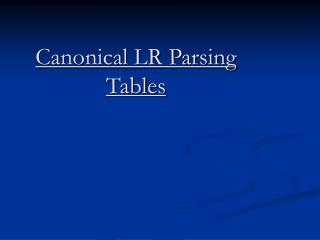DownloadDownload PresentationCanonical LR Parsing Tables

# Canonical LR Parsing Tables

Download Presentation## Canonical LR Parsing Tables

- - - - - - - - - - - - - - - - - - - - - - - - - - - E N D - - - - - - - - - - - - - - - - - - - - - - - - - - -
##### Presentation Transcript

1. Canonical LR Parsing Tables

2. Construction of the sets of LR (1) items • Input: An augmented grammar G’. • Output: The sets of LR (1) items that are the set of items valid for one or more viable prefixes of G’. • Method:Three functions are defined closure(), goto(), items()

3. Function closure (I) Begin Repeat For each item [A α. Bβ, a] in I, each production Bγ in G', and each terminal b in FIRST (βa) such that [B. γ, b] is not in I do add [B. γ, b] to I until no more sets of items can be added to I return I end;

4. functiongoto (I, X) begin let J be the set of items [AX. β, a] such that [AX β, a] is in I return closure (J) end;

5. procedure items (G') begin C := {closure ({S'.S, \$})}; repeat for each set of itemsI in C and each grammar symbol X such that goto (I,X) is not empty and not in C do add goto (IX) to C until no more sets of items can be added to C end;

6. EXAMPLE Consider the following augmented grammar:- S’  S S  CC C  Cc | d

7. The initial set of items is:- I0 : S’  .S, \$ S  .CC, \$ C  .Cc, c | d C  .d, c | d

8. We have next set of items as:- I1 : S’  S., \$ I2 : S  .Cc, \$ C  .Cc, \$ C  .d, \$ I3 : C  c.C, \$ C  .c C, c | d C  .d, \$

9. I4 : C  d. , c | d I5 : S  CC. , \$ I6 : C  c.C, \$ C  .c C, \$ C  .d, \$ I7 : C  d. , \$ I8 : C  c C. , c | d I9 : C  c C. , \$

10. Construction of the canonical LR parsing table • Input: An augmented grammar G’. • Output: The canonical LR parsing table functions action and goto for G’ • Method:

11. 1. Construct C= {I0, I1…... In}, the collection of sets of LR (1) items for G’. 2. State I of the parser is constructed from Ii. The parsing actions for state I are determined as follows : a) If [A  α. a β, b] is in Ii, and goto (Ii, a) = Ij, then set action [i, a] to “shift j.” Here, a is required to be a terminal. b) If [A  α., a] is in Ii, A ≠ S’, then set action [i, a] to “reduce A  α.” c) If [S’S., \$] is in Ii, then set action [i, \$] to “accept.”

12. If a conflict results from above rules, the grammar is said not to be LR (1), and the algorithm is said to be fail. 3. The goto transition for state i are determined as follows: If goto(Ii , A)= Ij ,then goto[i,A]=j. 4. All entries not defined by rules(2) and (3) are made “error.” 5. The initial state of the parser is the one constructed from the set containing item [S’.S, \$].

13. Submitted by: Tarun Chauhan 04CS1023